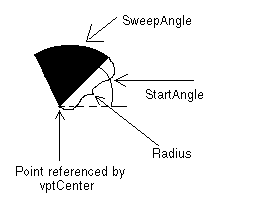﻿ VECTORPIE | Used Structures | Vector C API Help
In This Topic ▼

# VECTORPIE / VECTORCHORD

````typedef  struct  tagVECTORPIE `
`{ `
`   VECTOROBJECT  Object; `
`   VECTORPOINT  Point; `
`   VECTORPEN  Pen; `
`   VECTORBRUSH  Brush; `
`   L_DOUBLE  Radius; `
`   L_DOUBLE  StartAngle; `
`   L_DOUBLE  SweepAngle; `
`}  VECTORPIE,  *  pVECTORPIE,  VECTORCHORD,  *  pVECTORCHORD; ````

The VECTORPIE structure holds information about a vector arc object.

The VECTORCHORD structure holds information about a vector chord object.

## Members

Abstract object.

### VECTORPOINT Point

A VECTORPOINT structure that contains the vertex of a vector pie object, about which the pie section or chord sweeps.

### VECTORPEN Pen

VECTORPEN structure that contains information about the pen to use when drawing the vector pie or vector chord object.

### VECTORBRUSH Brush

VECTORBRUSH structure that contains information about the brush to use when drawing the vector pie or vector chord object.

### L_DOUBLE Radius

Length of the radius of the vector pie or vector chord object. This value is in logical units.

### L_DOUBLE StartAngle

The angle at which to begin the vector pie or vector chord. Values should be between 0 and 360 degrees. Values less than 0 and greater than 360 will be modified internally.

### L_DOUBLE SweepAngle

The angle through which to sweep the vector pie or vector chord. Values should be between 360 and +360 degrees. Positive angles will be drawn in a counter-clockwise direction from StartAngle. Negative angles will be drawn in a clockwise direction.

## Comments

nSize of the Object member must be the sizeof this structure.

nType of the Object member must be VECTOR_PIE or VECTOR_CHORD.

pVECTORPIE is a pointer to a VECTORPIE structure.

pVECTORCHORD is a pointer to a VECTORCHORD structure.

The diagram below gives a graphical representation of the Point, Radius, StartAngle and SweepAngle parameters.Help Version 21.0.2021.4.7
Products | Support | Contact Us | Intellectual Property Notices
© 1991-2021 LEAD Technologies, Inc. All Rights Reserved.